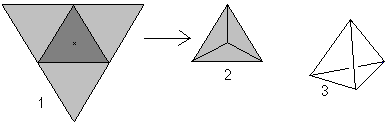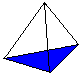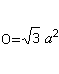Surface area of the tetrahedron in java

What is a Tetrahedron?Thanks mathematische-basteleien.de
A tetrahedron is a three-dimensional figure with four equilateral triangles. If you lift up three triangles (1), you get the tetrahedron in top view (2). Generally it is shown in perspective (3).

If you look at the word tetrahedron (tetrahedron means "with four planes"), you could call every pyramid with a triangle as the base a tetrahedron.
However the tetrahedron is the straight, regular triangle pyramid on this website.

Surface :.........The area of the base and the lateral faces form the surface O. There is O=4*A (triangle) = sqr(3)a².

Java version of the n-queens problem is given below:
/**
* To change this template, choose Tools | Templates
* and open the template in the editor.
*/
package area;

import java.util.Scanner;

/**
*
* @author ACHCHUTHAN
*/
public class Tetrahedron {

double s1, s2, s3;

Tetrahedron() {
Scanner input = new Scanner(System.in);
System.out.println("Enter side 1 length : ");
s1 = input.nextDouble();
System.out.println("Enter side 2 length : ");
s2 = input.nextDouble();
System.out.println("Enter side 3 length : ");
s3 = input.nextDouble();

}

public double getSurfaceArea() {
double s = (s1 + s2 + s3) / 2;
double a = Math.sqrt(s * (s - s1) * (s - s2) * (s - s3));
return (double)(4 * a);
}

public static void main(String args[]) {
Tetrahedron t = new Tetrahedron();
System.out.println("***SurfaceArea of Tetrahedron****" + t.getSurfaceArea());

}
}

Output of this program is given bellow :

run:
Enter side 1 length :
3
Enter side 2 length :
4
Enter side 3 length :
5
***SurfaceArea of Tetrahedron****24.0
BUILD SUCCESSFUL (total time: 4 seconds)